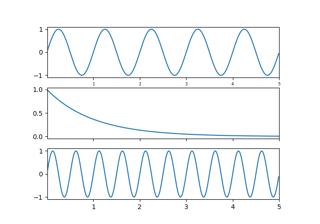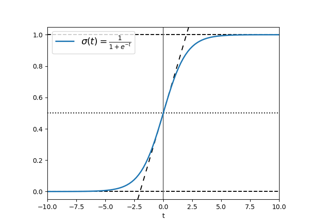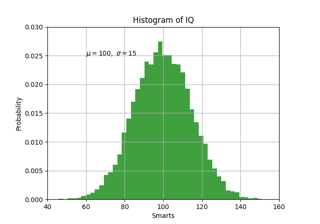# matplotlib.pyplot.xlim#

matplotlib.pyplot.xlim(*args, **kwargs)[source]#

Get or set the x limits of the current axes.

Call signatures:

```left, right = xlim()  # return the current xlim
xlim((left, right))   # set the xlim to left, right
xlim(left, right)     # set the xlim to left, right
```

If you do not specify args, you can pass left or right as kwargs, i.e.:

```xlim(right=3)  # adjust the right leaving left unchanged
xlim(left=1)  # adjust the left leaving right unchanged
```

Setting limits turns autoscaling off for the x-axis.

Returns:
left, right

A tuple of the new x-axis limits.

Notes

Calling this function with no arguments (e.g. `xlim()`) is the pyplot equivalent of calling `get_xlim` on the current axes. Calling this function with arguments is the pyplot equivalent of calling `set_xlim` on the current axes. All arguments are passed though.

## Examples using `matplotlib.pyplot.xlim`#Shared Axis

Shared AxisInfinite lines

Infinite linesPyplot Text

Pyplot TextFrame grabbing

Frame grabbingInteractive functions

Interactive functions